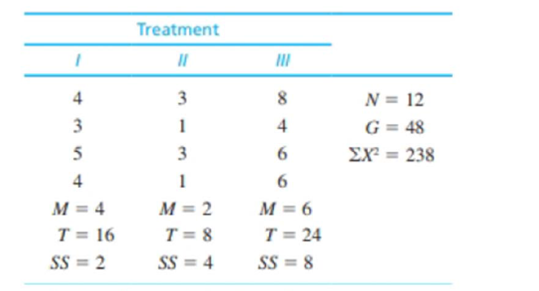Chapter 12, Problem 8PEssentials of Statistics for the B...

8th Edition
Frederick J Gravetter + 1 other
ISBN: 9781133956570

Solutions

Chapter
SectionEssentials of Statistics for the B...

8th Edition
Frederick J Gravetter + 1 other
ISBN: 9781133956570
Textbook Problem

The following data summarize the results from an independent-measures study comparing three treatment conditions.a. Calculate the sample variance for each of the three samples.b. Use an ANOVA with α = .05 to determine whether there are any significant differences among the three treatment means.

a.

To determine

To find: The sample variance for each of the three samples.

Explanation

Given info:

The data values and details are provided in the question.

 Treatment 1 Treatment 2 Treatment 3 N=12G=48∑X2=238 n=4 n=4 n=4 M=4 M=2 M=6 T=16 T=8 T=24 SS=2 SS=4 SS=8

Calculations:

The formula for sample variances is

s2=SSn1

For treatment 1:

s12=SS1n11 <

b.

To determine

To find: Whether there are any significant differences among the three treatments means using ANOVA with α=0.05

Still sussing out bartleby?

Check out a sample textbook solution.

See a sample solution

The Solution to Your Study Problems

Bartleby provides explanations to thousands of textbook problems written by our experts, many with advanced degrees!

Get Started

Construct a drawing of the fourth hexagonal number.

Mathematical Excursions (MindTap Course List)

Find the derivative of the function. y=234x

Single Variable Calculus: Early Transcendentals, Volume I

True or False: If converges absolutely, then it converges conditionally.

Study Guide for Stewart's Single Variable Calculus: Early Transcendentals, 8th